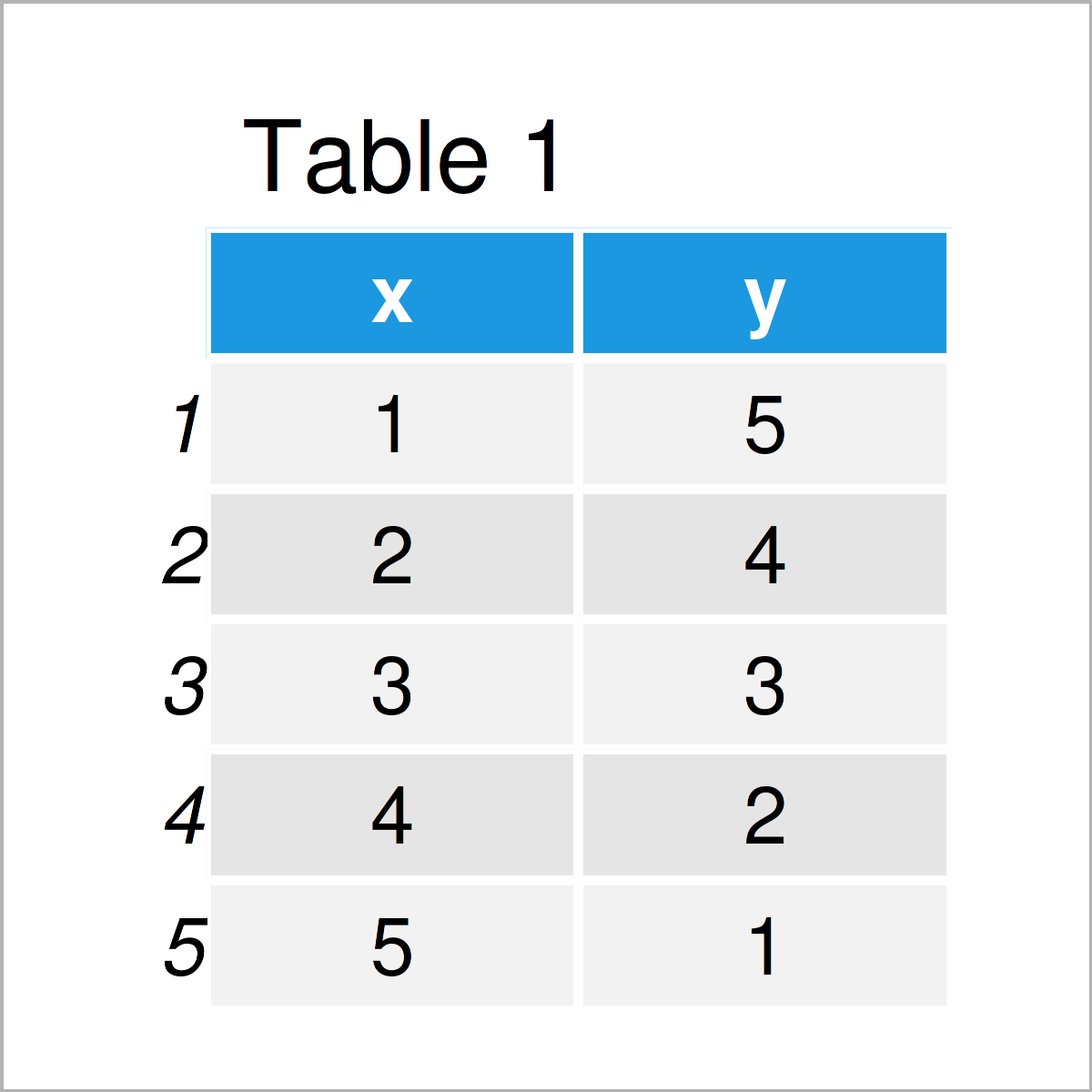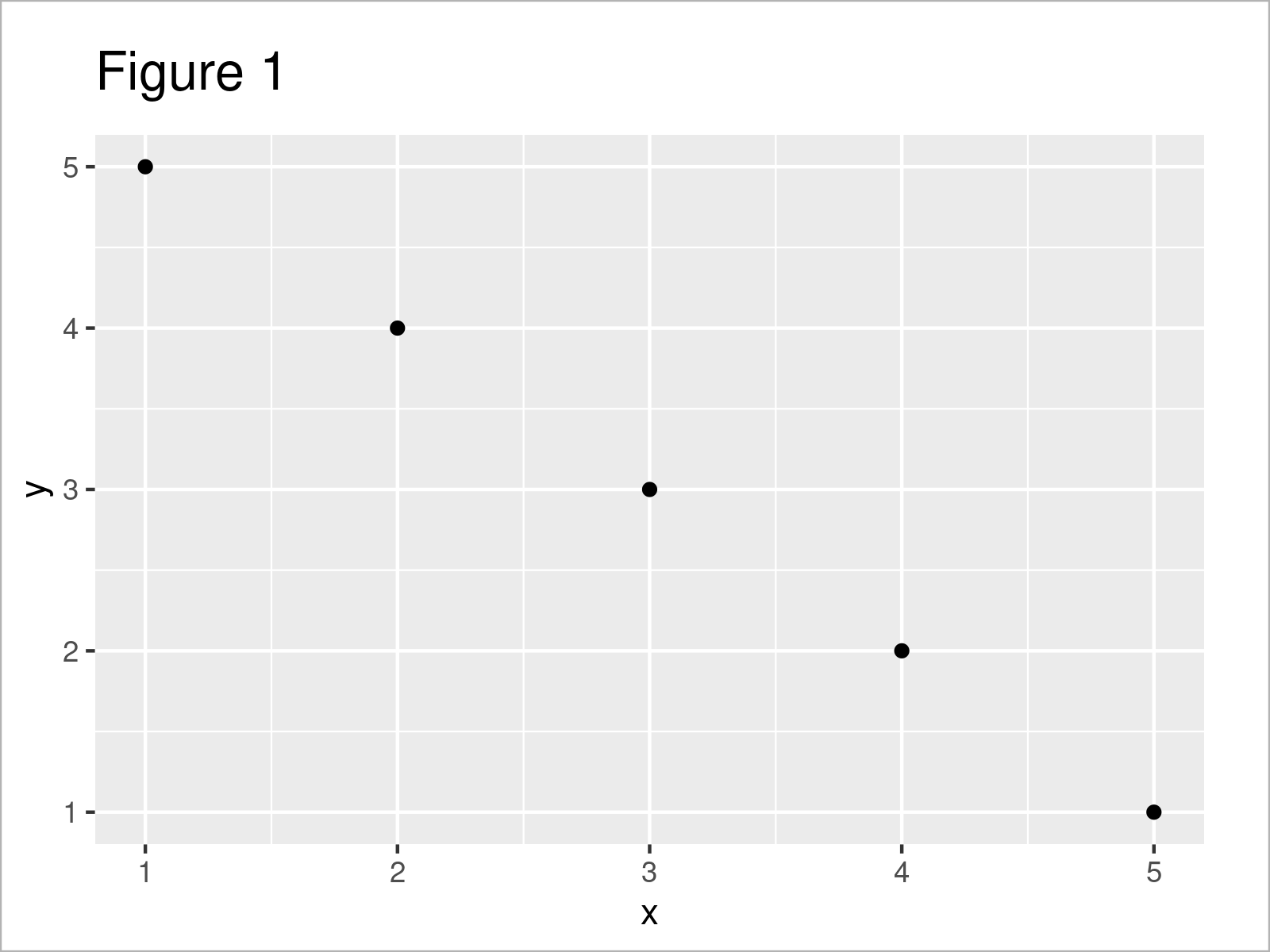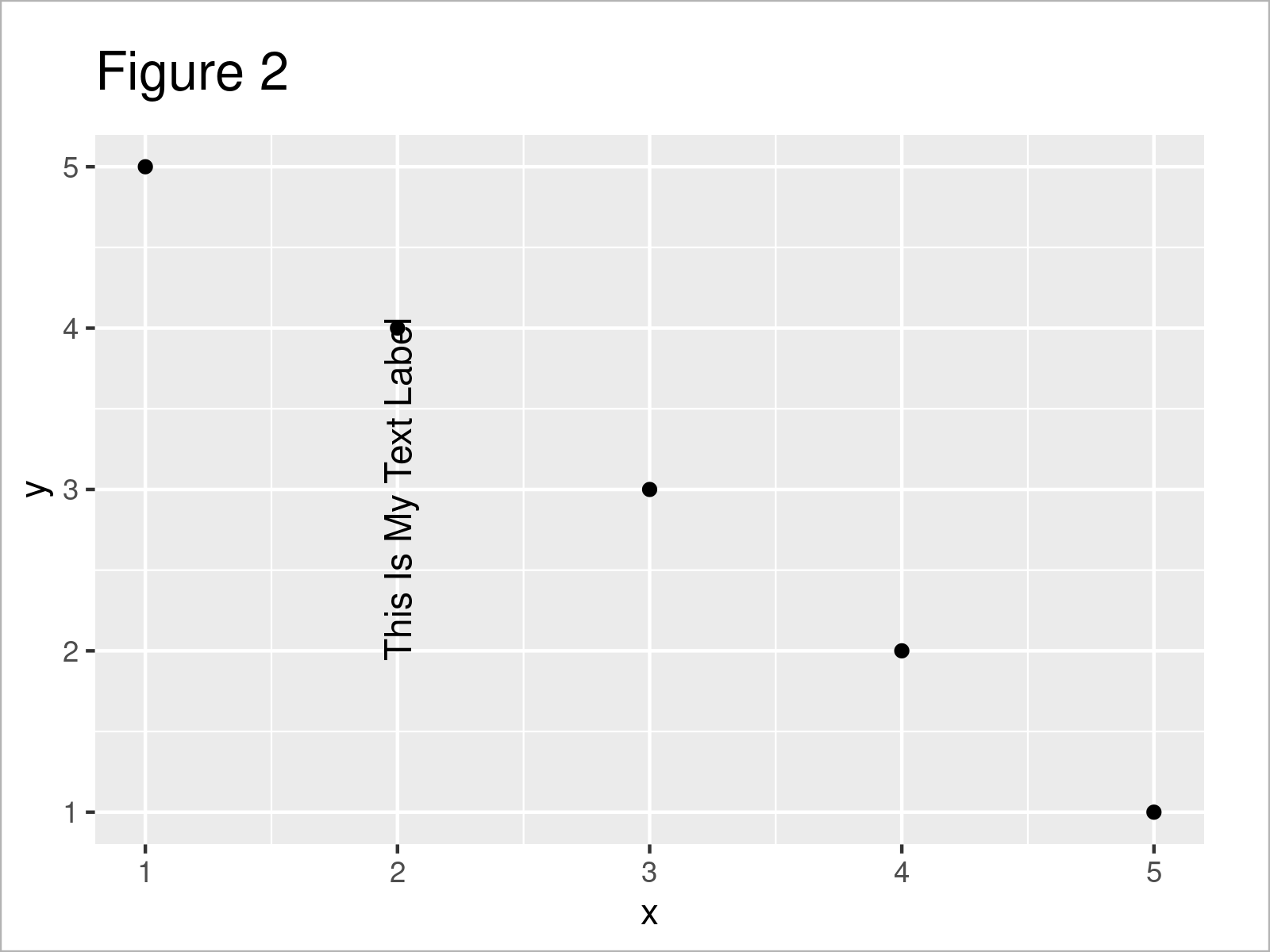# Rotate Annotated Text in ggplot2 Plot in R (Example)

This tutorial explains how to annotate a rotated text label to a ggplot2 graph in the R programming language.

Here’s the step-by-step process:

## Example Data, Add-On Packages & Default Plot

Consider the following example data.

```data <- data.frame(x = 1:5,         # Create example data
y = 5:1)
data                                # Print example data```Table 1 illustrates the output of the RStudio console and shows the structure of our example data – It contains five rows and two integer variables called “x” and “y”.

In order to draw our data using the ggplot2 package, we also need to install and load ggplot2:

```install.packages("ggplot2")         # Install ggplot2 package

As next step, we can draw our data:

```ggp <- ggplot(data, aes(x, y)) +    # ggplot2 plot without text label
geom_point()
ggp```As illustrated in Figure 1, we have managed to create a ggplot2 scatterplot without any additional text elements by running the previous R programming syntax.

## Example: Rotate Annotated Text in ggplot2 Plot Using angle Argument

This section illustrates how to add a text label with a certain degree of rotation to a ggplot2 plot.

For this, we can use the annotate function and and the angle argument of the annotate function. To the angle argument, we have to assign the degree of rotation that we want to use (i.e. 90 degree).

Consider the following R code:

```ggp +                               # Annotate rotated text label
annotate("text",
x = 2, y = 3,
label = "This Is My Text Label",
angle = 90)```As shown in Figure 2, the previous R code has created a ggplot2 with rotated text.

## Video & Further Resources

Would you like to learn more about the annotation of a rotated text element to a ggplot2 graph? Then I recommend having a look at the following video on my YouTube channel. In the video, I’m explaining the R syntax of this tutorial in R:

Please accept YouTube cookies to play this video. By accepting you will be accessing content from YouTube, a service provided by an external third party.If you accept this notice, your choice will be saved and the page will refresh.

Besides that, you might want to read the other articles which I have published on my website. A selection of tutorials about related topics such as ggplot2, graphics in R, and text elements can be found below.

In summary: In this article, I have illustrated how to add a rotated text label to a ggplot2 graphic in R programming. Please let me know in the comments section, if you have further questions.

Subscribe to the Statistics Globe Newsletter